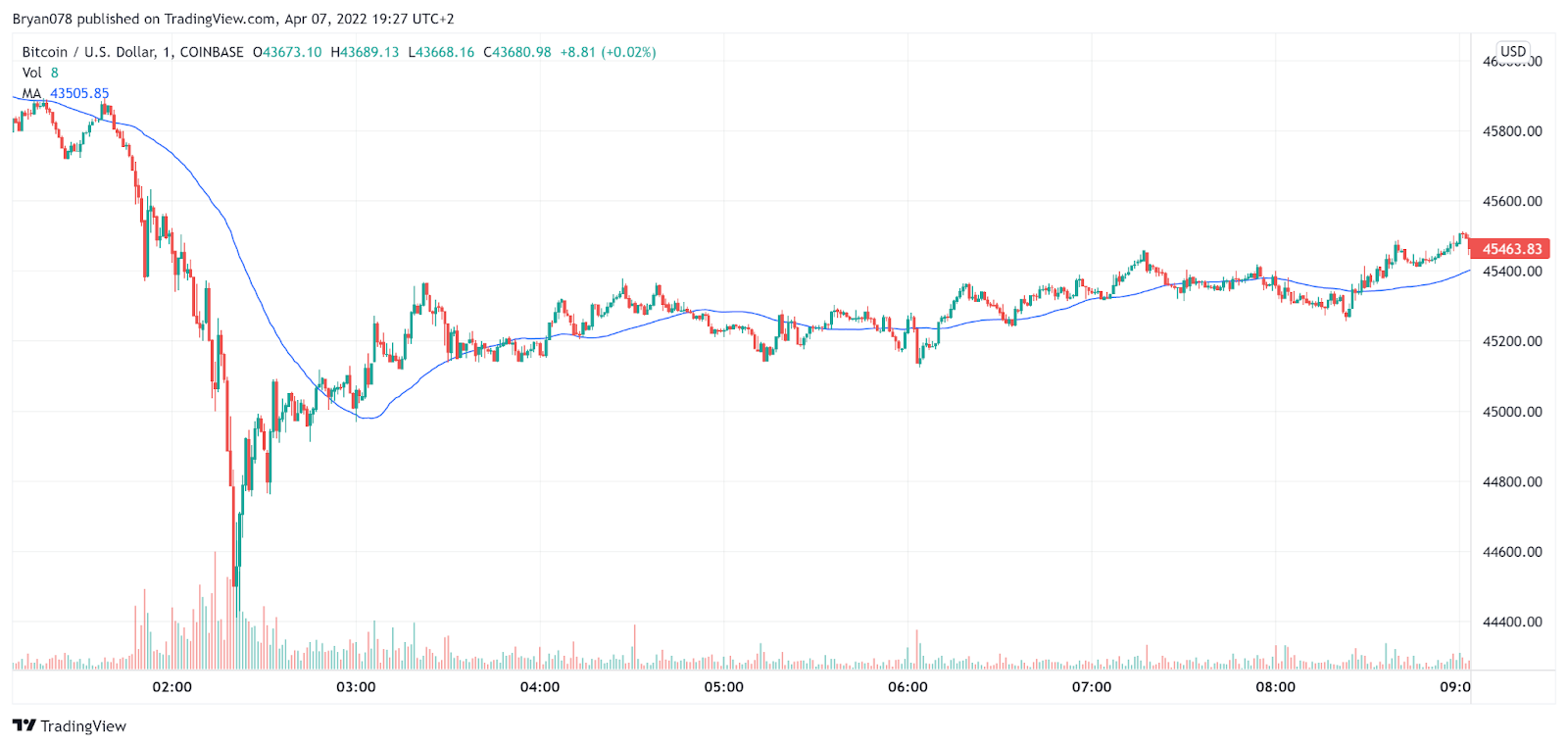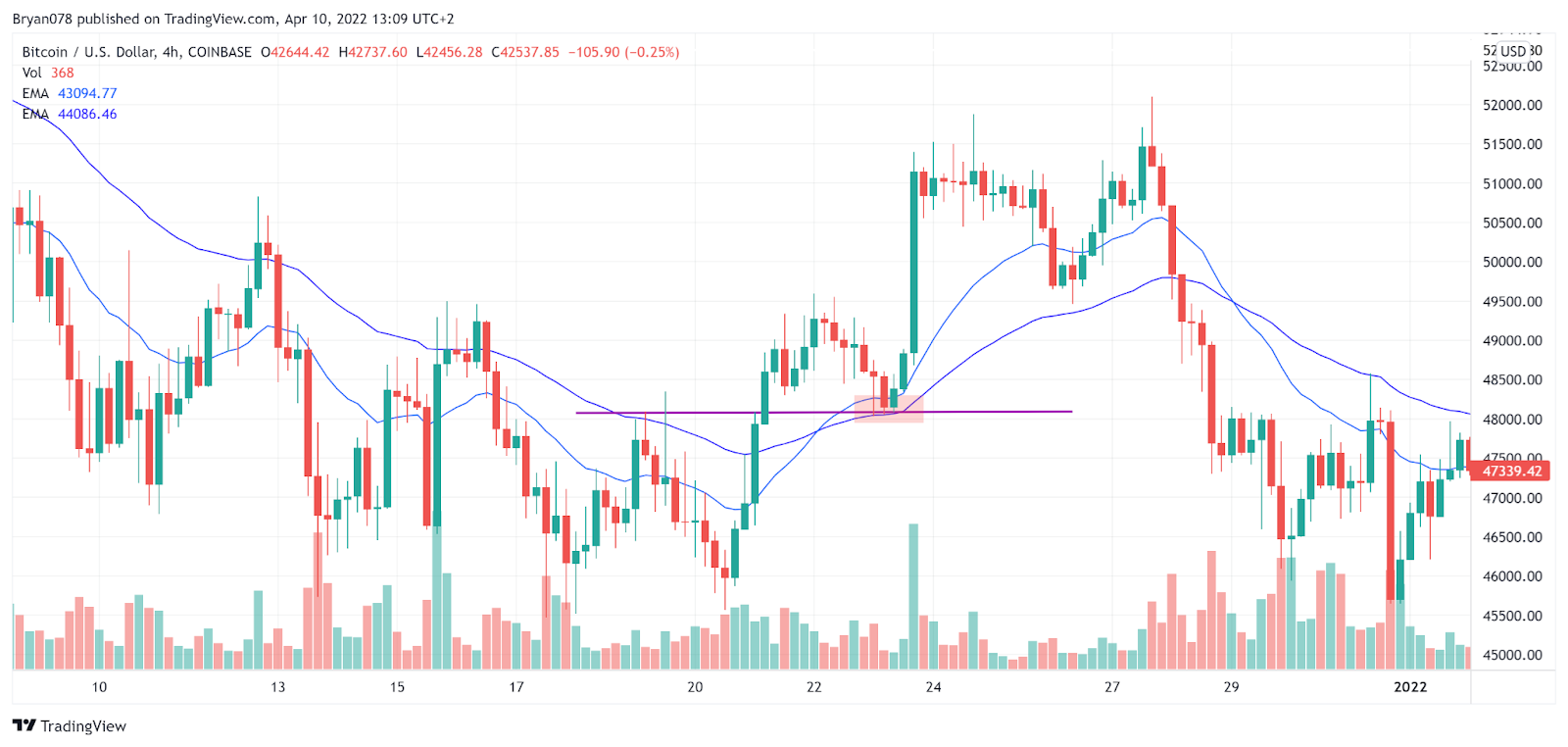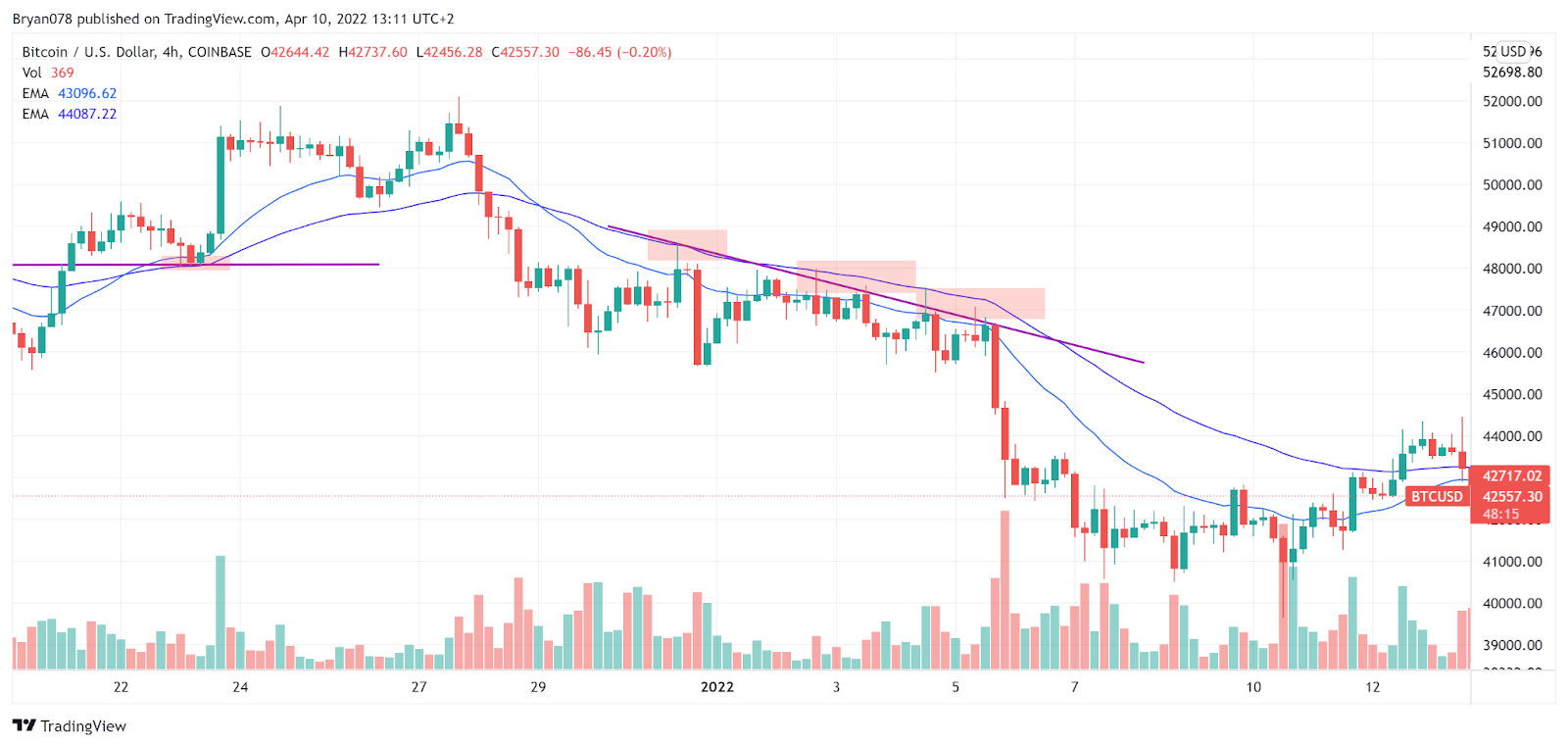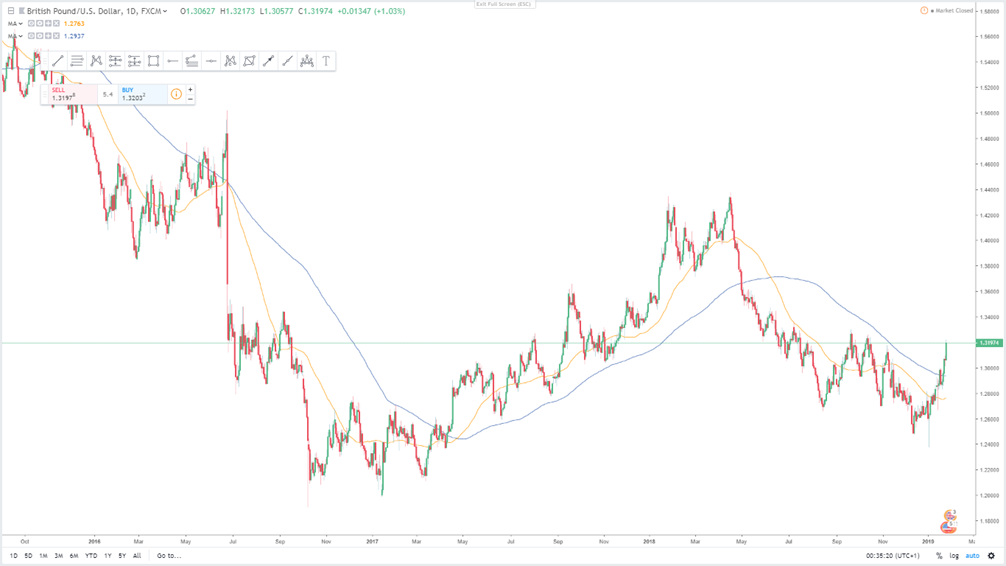This picture will be mintable soonBryan

## Moving Averages: Simple & Exponential

Moving averages are one of the most widely utilised trading indicators. Although they incorporate the idea that price tends to follow trends, they are timeframe-dependent and can be interpreted differently depending on the trading technique used. In today’s article, I’ll go over how these moving averages are calculated and more importantly, how you can apply moving averages strategies as a beginner trader.

## The Basics of Moving Averages (MAs)

A Moving Average (MA) is a popular technical analysis indicator that filters out “noise” from short-term price fluctuations. It is a trend-tracking indicator precisely because it is predicated on historical prices.

The Simple Moving Average (SMA), which provides clarity over a set number of periods, and the Exponential Moving Average (EMA), which focuses more on current prices, are the most common and extensively used MAs in trading. The MAs have traditionally been used to determine trend direction, support, and resistance zones.

MA’s are known to lag behind price activity. The longer the MA, the more considerable lag. Hence, this is why we refer to them as lagging indicators. They don’t tell us anything about present price action but give us more information about the market’s broad direction and tendency.

These indicators have the advantage of being applicable to all timeframes. In other words, regardless of the timeframe you choose (for example, 1h, 4h, or even 1min), the 50MA indicator will automatically adjust to take the 50 most recent hours for the 1h chart or the 50 most recent minutes for the 1min minute chart.

An interesting note is that bigger MAs on smaller timescales can likewise be used as benchmarks for smaller MAs on larger timeframes. Taking the 200EMA on the 1h timeframe, for example, correlates to the 50EMA on the 4h timeframe. Although the math is a bit of a mental exercise, it is a handy trick that may be applied over a variety of timeframes in order to conceptualise EMAs.

To better understand the use-cases of moving averages, let’s first explore SMAs. SMAs, as the name suggests, are calculated using a simplistic calculation that does not consider the weights of data/price points. They are derived using the following formula:

• SMA = ( Sum ( Price, n ) ) / n
• Where: n = Time Period

This brief calculation helps determine the average price for the respective number of days. The initial data point for a 10-day MA would be calculated using the closing price of the first 10 days prior to the closure of the current trading day.The 1min 50MA indicator automatically takes the 50 most recent minutes (or the 50 most recent hours if you’re looking at the hourly chart). Timeframes are critical in determining how traders capitalize on this indicator. They also explain how the graph is generated over a period of time since price movement throughout that time interval causes it to become a ‘moving’ average. As such, the SMA is updated every time an increment of the selected time frame passes, as can be seen in the graph above.

## What is an Exponential Moving Average (EMA)?

The exponential moving average (EMA) is a technical chart indicator that shows how the price of an investment (such as a stock, commodity, or cryptocurrency) has changed over time. The EMA is a weighted moving average (WMA) that attaches more weight or importance to the latest price data. Although like the simple moving average (SMA), the EMA is used to analyse price trends over time, its higher price sensitivity causes it to lag to a lesser degree.

Each time an increment of the selected time frame passes, the EMA is recalculated using the following formula:

EMAToday=(ValueToday∗(1+DaysSmoothing))+EMAYesterday∗(1−(1+DaysSmoothing))

Therefore, as is done with the SMA, the first measurement would be removed and replaced by the next and newest measurement (hence why it moves along with the selected time frame).

### What are the main differences between their use cases?

In brief, the EMA is supposed to ameliorate the idea of an SMA by assigning more weight to recent price data, which is often seen to be more important than older data for traders. However, let’s go over some of the main similarities and differences:

• Similarities
• SMAs and EMAs both aid traders in determining the market’s broad direction.
• When calculating the average, both use the same number of data points. The 1h 50EMA and 50SMA both use the previous 50H as data points.
• SMAs and EMAs can be utilised as support and resistance markers.
• Differences
• Weighted MAs are called EMAs while non-weighted or homogenous weights per data points are called SMAs.
• Because EMAs use more recent data, they are often more price sensitive.
• Due to its sensitivity, it has a shorter lag-time than SMAs for starting and exiting trades based on this gauge than its simple equivalent.

#### Support & Resistance

While MAs are sufficient, they can also act as support and resistance. See the article on onXRP about support and resistance:

##### Support
• The Purple line represents the resistance (zone/line)
• The continuous Blue and Dark Blue lines represent the 20EMA and 50EMA respectively.

Here, the chart signals a good opportunity for traders because the 20EMA is above the 50EMA, the price is bouncing off the 50EMA on a large time frame (4h) AND it is bouncing off a previous resistance (the red box). Such opportunities can be fruitful when capitalized. However, this is by no means financial advice.##### Resistance
• The straight Purple line represents the resistance (zone/line)
• The continuous Blue and Dark Blue lines represent the 20EMA and 50EMA respectively.

In this case, the chart signals a good opportunity because the 20EMA is below the 50ema, the price cannot break through the EMAs after falling AND it is rejecting previous resistance levels in the red boxes. Again, capitalizing on such opportunities can be fruitful, but this is at one’s own discretion.Both the SMA and EMA can be used as an indicator to open a trade, either a short or long trade.

#### Mean Reversion

Mean reversion is another strategy used by big players like market makers. Since EMAs and SMAs are real-time reflections of buy and sell orders, prices often fall or surge spontaneously through them (fall through support, breakthrough resistance). However, in such instances, the price has a propensity to ‘revert’ back toward the EMAs if it falls or rises far enough from these indicators. Traders (usually scalpers) trade on the strength of the rebound using leverage to capitalize on a fast price ‘reversion’. Although this can be a very profitable strategy, it takes years to master. This is not financial advice and should therefore only be used at one’s discretion.

#### Determining the Trend

Because it comprises the prices from the previous 200 days, a 200-day MA will have a far greater lag than a 20-day MA. Why? The average is calculated using ten times more points than the original 20 data points.

When it comes to trading, which MA to utilise is dependent on the size of the selected timeframe. Larger MAs are better for long-term investors, whereas lower MAs are better for short-term traders (think scalpers and day traders vs. HODLers and Swing traders).

Although this is the popular view, both investors and traders use the 50-day and 200-day MAs to determine the market’s overall trend. This is because MAs can provide key trading indicators. A rising MA suggests that prices are growing, while a falling MA suggests the opposite. A bullish crossover occurs when a small MA passes over a large MA, confirming upward momentum (see chart). Conversely, when a smaller MA crosses below a larger MA, a bearish crossover is confirmed which conveys a shift toward a more bearish trend (see chart).

The 5 and 13EMA are also two vital moving averages used to determine short-term market direction. The price (candles) is in a solid upward tendency if it is above these EMAs and pointing upward. Contrarily, the price is deemed to be in a downward trend if it falls below the EMAs and the 5EMA crosses the 13EMA in a downward fashion. If the price ends up being rejected anew, the trend has reversed.• Orange: 50sma
• Blue: 150sma

There is an uptrend if the sma50 is above the sma150 and a downtrend if the 50MA is below the 150SMA. It can be seen clearly in the above chart. At the arrows, when a crossover occurs, the trend shifts.

## Conclusion

Technical analysis, a form of investing that aims to understand and profit from the price movement patterns of securities and indices, makes extensive use of moving averages. The SMA is the most straightforward moving average to construct. It’s just the average price for a given time. Because it is shown on the chart bar by bar, generating a line that moves along the chart as the average value changes, the average is dubbed “moving.” EMAs are popular because they give recent prices more weight and lag less than other averages. Eight different EMA lines, varying in duration from a few days to numerous months, are used in classic moving average ribbon examples, which will be discussed at a later stage.